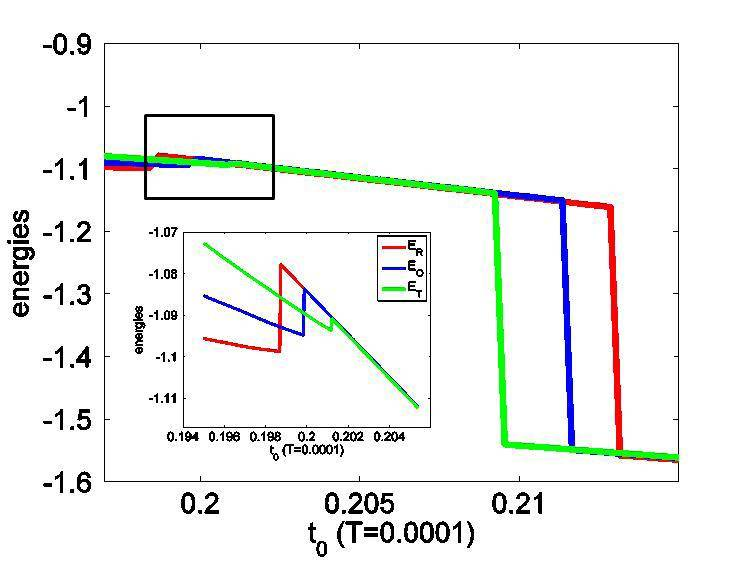# Is this free energy possible ?

hiyok
hi,
I'm studying a system using self-consistent mean field theory. And I have obtained its free energy, which however looks a bit unusual in that it bears a steep (almost abrupt) rise across the phase transition point (see the figure). This feature brought about fierce questioning from my boss. He said a free energy should not have such steep rise. But I think it is possible: around the transition point, the order parameter decreases very fast, and hence the potential energy, which is proportional to the square of the order parameter, should increase fast accordingly.
How do you think, my friends ?kanato
What is your definition of your free energy? The Helmholtz free energy is generally a monotonic function, dF = -S dT - P dV since S > 0 and P > 0 for all but very unusual systems.

Also, what is your horizontal axis? Heck, you should state what your Hamiltonian is too while you're at it.

hiyok
I'm considering a quantum phase transition, so the free energy is simply the ground state energy. The model is the so-called '8-site' model. t0 is the tunneling energy between adjacent sites.

kanato

Can you show analytically that dE/dt0 doesn't have a definite sign?

hiyok
Each line stands for the energy of a phase, as indicated by the R, T and O. I try to map out the phase diagram by comparing the energies. Unfortunately, I was not able to obtain analytical dE/dt0. Accompanying the rapid rise in energy, I observed a rapid reduction in the order parameter. Have you ever seen a physical system with similar changes in energy across phase transitions ?

kanato
If each line is the energy of a phase, why are there discontinuities at all?

Can you break the energies into kinetic and potential parts and see if the change is entirely from the potential part?

hiyok
(1) Sorry, I should not say each line represents the energy of a phase. More accurately, each line represents the energy of a configuration, and each configuration might admit two phases depending on the t0. By configuration, I mean the direction of the order parameter: for example, in the R-configuration, the order parameter is fixed along [1,1,1] direction, while its magnitude is variable.

(2) I believe the energy rise should be entirely due to potential energy. Across the transition point, the order parameter decreases, implying mobilization of the particle and hence kinetic energy lowering. Thus, the energy rise should be totally from potential energy.

kanato
Well it might help to convince your boss if you can show plots of kinetic and potential energy separately, as well as the magnitude of the order parameter and show that it correlates with the rise in your free energy. If you can find a way to show that the energy is not a monotonic function of the parameter t0 that might help also.

hiyok
Good suggestion.
Thanks for the discussions.

Homework Helper
I'm a bit confused, so let me get some details straight. Is the following correct?

1) The R, T and O labels represent configurations, each of which has two phases.
2) Each configuration exhibits a phase transition near t0 = 0.198 to 0.202, at which point the order parameter's magnitude drops.

The first point of confusion for me is that you call the rise in energy near t0 = 0.2 a phase transition, and you say each configuration admits two phases. What then is the sharp drop in free energy beyond t0 = 0.21? Are they not associated with any phase transition? They look like they ought to be. They look like much sharper drops than the slight rises. So, are there only two phases, in which case if the transition is the slight rise I would like to know why the drop appears to sharp, or are there two phase transitions, meaning three phases?

The second thing I am wondering is, for a given configuration, if the slight rise is really a phase transition, then when the phase transition occurs does the order parameter vanish completely? If not, why not? Order parameters are supposed to be quantities that are non-zero in ordered phases and zero in disordered phases. At very least, some component of the order parameter should vanish, no? If your order parameter is vector-valued, and the direction of the value determines which configuration you're in, and is fixed, then if each configuration only has two phases I would expect the magnitude to be zero in one phase and non-zero in another. Can you tell me why this is not the case in your system? Is it simply that your order parameter isn't technically an order parameter?

Finally, do you know whether the phase transition is continuous or discontinuous? If the transition is discontinuous and the free energy depends on the order parameter, then naturally the free energy will exhibit a jump at the transition.

hiyok
@Mute:
(1) "They look like much sharper drops than the slight rises. So, are there only two phases, in which case if the transition is the slight rise I would like to know why the drop appears to sharp, or are there two phase transitions, meaning three phases?"

Yes, exactly.

(2) In line with (1), the order parameter drops to a lower value across the first phase transition point and diminishes further to zero across the second transition point.

(3) I'm not quite sure (but I tend to believe) if the first transition is continuous, but the second one is continuous (although very sharp). And the free energy depends on order parameter.